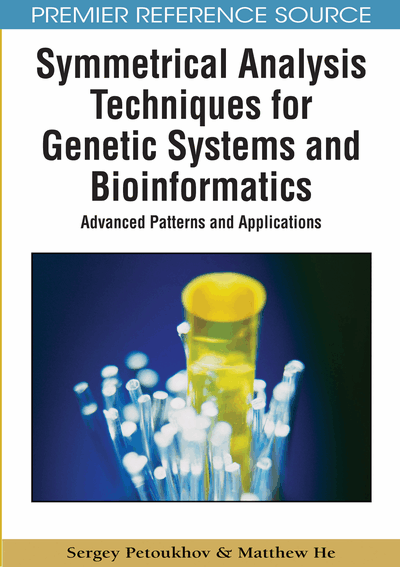# Numeric Genomatrices of Hydrogen Bonds, the Golden Section, Musical Harmony, and Aesthetic Feelings

Sergey Petoukhov (Russian Academy of Sciences, Russia) and Matthew He (Nova Southeastern University, USA)
DOI: 10.4018/978-1-60566-124-7.ch004
Available
\$37.50
No Current Special Offers

## Abstract

This chapter is devoted to a consideration of the Kronecker family of the genetic matrices, but in the new numerical form of their presentation. This numeric presentation gives opportunities to investigate ensembles of parameters of the genetic code by means of system analysis including matrix and symmetric methods. In this way, new knowledge is obtained about hidden regularities of element ensembles of the genetic code and about connections of these ensembles with famous mathematical objects and theories from other branches of science. First of all, this chapter demonstrates the connection of moleculargenetic system with the golden section and principles of musical harmony.
Chapter Preview
Top

## Introduction And Background

Till this moment we analyzed the symbolic genetic matrices. Now we begin to analyze numeric genetic matrices, which are produced from the symbolic genomatrices. What are initial reasons to pay attention to numeric genomatrices?

The previous chapters demonstrated that the Kronecker product of matrices is useful for analysis of genetic code and is adequate for its structure. But the Kronecker product possesses some distinctive properties, which are connected with eigenvalues of matrices: eigenvalues of the Kronecker product A⊗B for two matrices A and B, which have their eigenvalues αi and βk, are equal to the products αik of these eigenvalues. This property gives an additional opportunity to introduce the notion of the Kronecker product into mathematics (Bellman, 1960). But if eigenvalues are so important for the theme of Kronecker products, one should investigate numeric genomatrices, which possess eigenvalues (symbolic matrices do not possess eigenvalues).

Also we will try to investigate genetic sequences from the viewpoint of the theory of digital signal processing. This theory presents a signal in the form of a sequence of its numeric values in points of references. Discrete signals are interpreted as vectors of multi-dimensional spaces: a value of the signal in each time (a moment of reference) is interpreted as the value of one of the coordinates of multi-dimensional space of signals (Trahtman, 1972). The theory of discrete signals processing is the geometrical science about multidimensional spaces in some extent. The number of dimensions of such space is equal to the quantity of moments of references for the signal. Appropriate metric notions and other necessary things for providing the reliability, velocity and economy of information transfer are introduced in these multi-dimensional vector spaces. For example, important information notions of the energy and of the power of a discrete signal are correspondingly the square of the length of the vector-signal and the same square of the length of the vector-signal, which is divided by the number of dimensions. Various signals and their ensembles are compared as geometrical objects of such metric multi-dimensional spaces.

These methods underline technologies of signal intelligence and pattern recognition, detections and corrections of information mistakes, artificial intellect and robot learning, etc. If we wish to use the methods of the theory of discrete signals processing for analyzing the genetic structures, we should learn to turn from the symbolic genetic matrices and genetic sequences to their numeric analogies.

The method, which is utilized in this book for such a turn, replaces the letter symbols A, C, G, U(T) of the genetic alphabet by quantitative parameters of these nitrogenous bases, which determine their physical-chemical role (Petoukhov, 2001a). First of all, these symbols are replaced in this chapter by numbers of the hydrogen bonds, which are suspected long ago as important participants of transferring of genetic information. Each molecular element of the genetic code is a component part of a harmonic system of genetic coding. Its molecular parameters are coordinated with quantitative parameters of other elements of this system. Quantitative characteristics of separate elements should be investigated as a part of the set of quantitative characteristics of system ensemble of elements. The matrix approach is known in science long ago as very effective for system investigations, for example, in the fields of quantum mechanics, physics of elementary particles, etc. In the field of matrix genetics, this approach unites parameters of a set of separate elements not only in a general matrix, but in the whole family of genetic matrices, which embraces sets of multiplets of different lengths (Figure 3 of Chapter 1). In this way hidden connections between parameters of separate parts of the united genetic system can be revealed together with their relations to famous physical and mathematical constants and other objects.

## Complete Chapter List

Search this Book:
Reset